# High School Math : Radius

## Example Questions

### Example Question #82 : Circles

How many times greater is the area of a circle with a radius of 4in., compared to a circle with a radius of 2in.?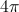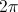Explanation:

The area of a circle can be solved using the equation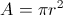The area of a circle with radius 4 is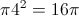while the area of a circle with radius 2 is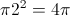.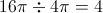### Example Question #83 : Circles

What is the area of a circle whose diameter is 8?

32π

64π

12π

8π

16π

16π

Explanation: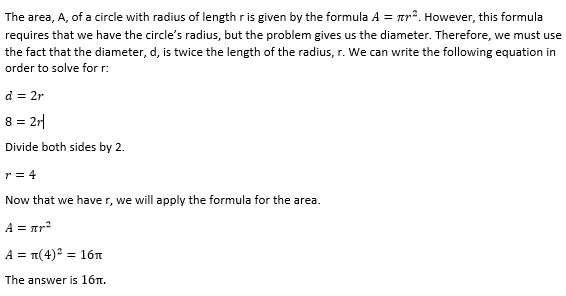### Example Question #31 : How To Find The Area Of A Circle

In the following diagram, the radius is given. What is area of the shaded region?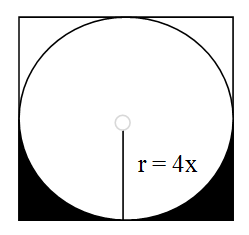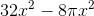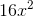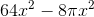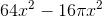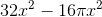Explanation:

This question asks you to apply the concept of area in finding both the area of a circle and square. Since the cirlce is inscribed in the square, we know that its diameter (two times the radius) is the same length as one side of the square. Since we are given the radius,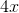, we can find the area of both the circle and square.

Square: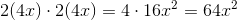This gives us the area for the entire square.

The bottom half of the square has area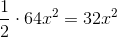.

Now that we have this value, we must find the area that the circle occupies. The area of a circle is given by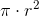.

So the area of this circle will be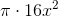.

The bottom half of the circle has half that area: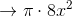Now that we have both our values, we can subtract the bottom half of the circle from the bottom half of the square to give us the shaded region: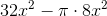### Example Question #85 : Circles

In a large field, a circle with an area of 144π square meters is drawn out. Starting at the center of the circle, a groundskeeper mows in a straight line to the circle's edge. He then turns and mows ¼ of the way around the circle before turning again and mowing another straight line back to the center. What is the length, in meters, of the path the groundskeeper mowed?

24π

12 + 36π

12 + 6π

24 + 36π

24 + 6π

24 + 6π

Explanation:

Circles have an area of πr2, where r is the radius. If this circle has an area of 144π, then you can solve for the radius:

πr2 = 144π

r 2 = 144

r =12

When the groundskeeper goes from the center of the circle to the edge, he's creating a radius, which is 12 meters.

When he travels ¼ of the way around the circle, he's traveling ¼ of the circle's circumference. A circumference is 2πr. For this circle, that's 24π meters. One-fourth of that is 6π meters.

Finally, when he goes back to the center, he's creating another radius, which is 12 meters.

In all, that's 12 meters + 6π meters + 12 meters, for a total of 24 + 6π meters.

### Example Question #86 : Circles

Two concentric circles have circumferences of 4π and 10π.  What is the difference of the radii of the two circles?

3

5

6

7

4

3

Explanation:

The circumference of any circle is 2πr, where r is the radius.

Therefore:

The radius of the smaller circle with a circumference of 4π is 2 (from 2πr = 4π).

The radius of the larger circle with a circumference of 10π is 5 (from 2πr = 10π).

The difference of the two radii is 5-2 = 3.

### Example Question #87 : CirclesIn the figure above, rectangle ABCD has a perimeter of 40. If the shaded region is a semicircle with an area of 18π, then what is the area of the unshaded region?

336 – 36π

96 – 18π

336 – 18π

96 – 36π

204 – 18π

96 – 18π

Explanation:

In order to find the area of the unshaded region, we will need to find the area of the rectangle and then subtract the area of the semicircle. However, to find the area of the rectangle, we will need to find both its length and its width. We can use the circle to find the length of the rectangle, because the length of the rectangle is equal to the diameter of the circle.

First, we can use the formula for the area of a circle in order to find the circle's radius. When we double the radius, we will have the diameter of the circle and, thus, the length of the rectangle. Then, once we have the rectangle's length, we can find its width because we know the rectangle's perimeter.

Area of a circle = πr2

Area of a semicircle = (1/2)πr2 = 18π

Divide both sides by π, then multiply both sides by 2.

r2 = 36

Take the square root.

r = 6.

The radius of the circle is 6, and therefore the diameter is 12. Keep in mind that the diameter of the circle is also equal to the length of the rectangle.

If we call the length of the rectangle l, and we call the width w, we can write the formula for the perimeter as 2l + 2w.

perimeter of rectangle = 2l + 2w

40 = 2(12) + 2w

Subtract 24 from both sides.

16 = 2w

w = 8.

Since the length of the rectangle is 12 and the width is 8, we can now find the area of the rectangle.

Area = l x w = 12(8) = 96.

Finally, to find the area of just the unshaded region, we must subtract the area of the circle, which is 18π, from the area of the rectangle.

area of unshaded region = 96 – 18π.

The answer is 96 – 18π.

### Example Question #88 : Circles

Consider a circle centered at the origin with a circumference of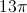. What is the x value when y = 3? Round your answer to the hundreths place.

5.778

10.00

5.8

5.77

5.77

Explanation:

The formula for circumference of a circle is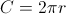, so we can solve for r: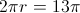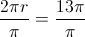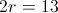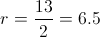We now know that the hypotenuse of the right triangle's length is 13.5. We can form a right triangle from the unit circle that fits the Pythagorean theorem as such: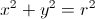Or, in this case: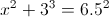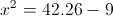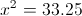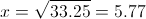### Example Question #89 : Circles

What is the radius of a circle with a circumference of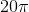?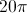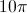Explanation:

To find the radius of a circle given the circumference we must first know the equation for the circumference of a circle which is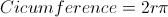Then we plug in the circumference into the equation yielding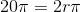We then divide each side bygiving us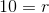The answer is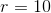.

### Example Question #1 : How To Find The Length Of A Radius

A circle has an area of 36π inches. What is the radius of the circle, in inches?

6

18

9

36

6

Explanation:

We know that the formula for the area of a circle is πr2. Therefore, we must set 36π equal to this formula to solve for the radius of the circle.

36π = πr2

36 = r2

6 = r

### Example Question #3 : How To Find The Length Of A Radius

Circle X is divided into 3 sections: A, B, and C. The 3 sections are equal in area. If the area of section C is 12π, what is the radius of the circle?Circle X

6

7

√12

4

6

Explanation:

Find the total area of the circle, then use the area formula to find the radius.

Area of section A = section B = section C

Area of circle X = A + B + C = 12π+ 12π + 12π = 36π

Area of circle =  where r is the radius of the circle

36π = πr2

36 = r2

√36 = r

6 = r